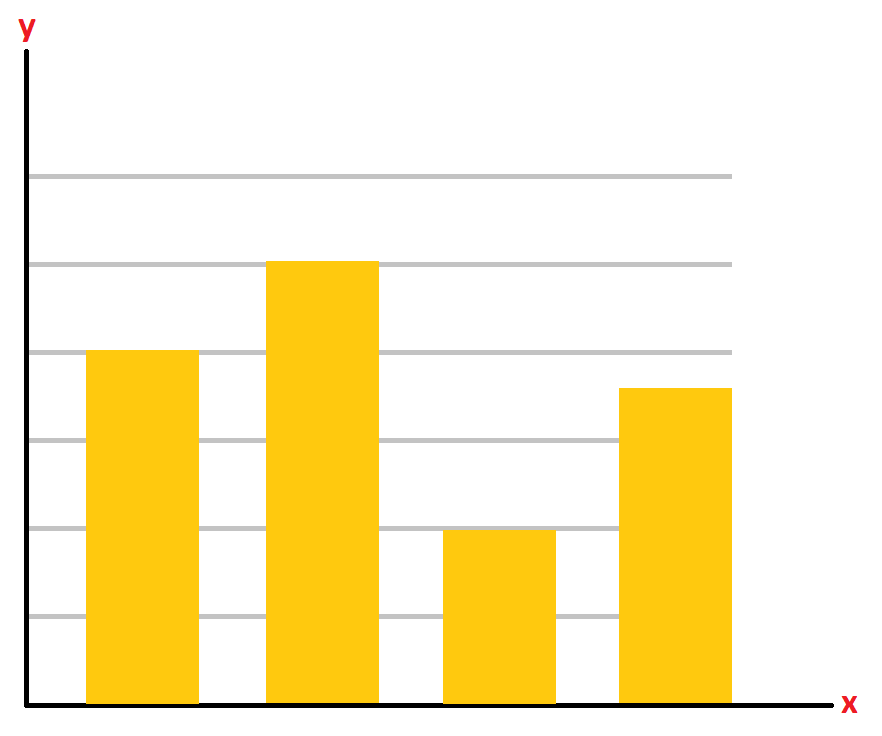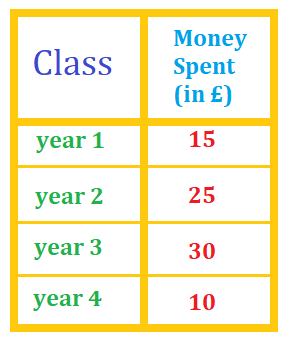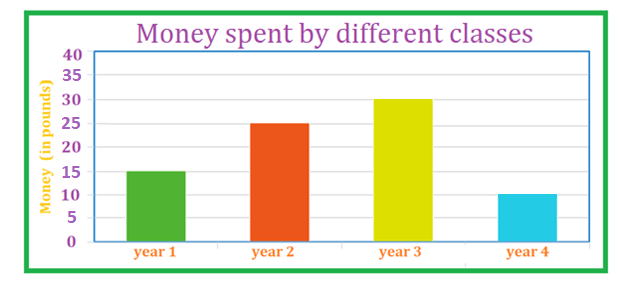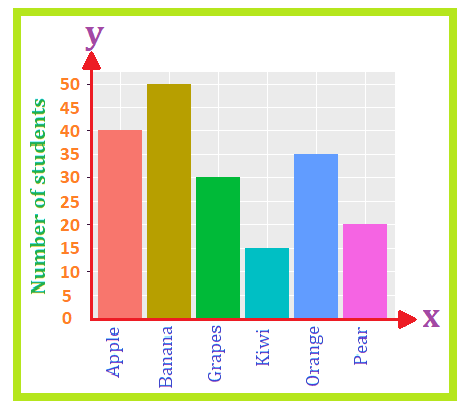# Math > Year 3

## Interpreting and Presenting data in bar charts

Bar Chart:
A bar graph is a graph in which the information (data) is represented by using bars of different heights.

A bar chart consists of two axis - namely vertical axis (y-axis) and the horizontal axis (x-axis).

Example-How to represent the data in a bar graph?

Let’s learn with the help of an example.

Represent the data given in the table below in a bar chart.Step 1: Give the title of the bar graph.

Step 2: Draw the horizontal axis (x-axis) and vertical axis (y-axis).

Step 3: Now label the horizontal axis and the vertical axis.

Step 4: Make rectangular bars with equal gaps for each category and give height to their respective numbers.

Step 5: The bar graph is ready, observe the height of rectangular bars to compare.How to interpret the bar graph?

Let’s learn with the help of an example.

The bar graph given below represents the favourite fruits of 190 students.Question 1: Which fruit is liked by the least number of students?

We can see that the rectangular bar of the smallest height represents the least number of students.

Question 2: How many students like oranges?The bar representing orange lies exactly between 30 and 40 i.e. 35.

35 students like oranges.

//